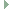OGRE  13.6 Object-Oriented Graphics Rendering Engine
Ogre::EdgeData Class Reference

This class contains the information required to describe the edge connectivity of a given set of vertices and indexes. More...

#include <OgreEdgeListBuilder.h>Inheritance diagram for Ogre::EdgeData:

## Classes

struct  Edge
Edge data. More...

struct  EdgeGroup
A group of edges sharing the same vertex data. More...

struct  Triangle
Basic triangle structure. More...

## Public Types

typedef std::vector< EdgeGroupEdgeGroupList

typedef std::vector< EdgeEdgeList

typedef aligned_vector< Vector4TriangleFaceNormalList
Array of 4D vector of triangle face normal, which is unit vector orthogonal to the triangles, plus distance from origin. More...

typedef std::vector< char > TriangleLightFacingList
Working vector used when calculating the silhouette. More...

typedef std::vector< TriangleTriangleList

## Public Member Functions

EdgeData ()

EdgeDataclone ()

void log (Log *log)
Debugging method. More...

void updateFaceNormals (size_t vertexSet, const HardwareVertexBufferSharedPtr &positionBuffer)
Updates the face normals for this edge list based on (changed) position information, useful for animated objects. More...

void updateTriangleLightFacing (const Vector4 &lightPos)
Calculate the light facing state of the triangles in this edge list. More...

## Public Attributes

EdgeGroupList edgeGroups
All edge groups of this edge list. More...

bool isClosed
Flag indicate the mesh is manifold. More...

TriangleFaceNormalList triangleFaceNormals
All triangle face normals. More...

TriangleLightFacingList triangleLightFacings
Triangle light facing states. More...

TriangleList triangles
Main triangles array, stores all triangles of this edge list. More...

## Detailed Description

This class contains the information required to describe the edge connectivity of a given set of vertices and indexes.

This information is built using the EdgeListBuilder class. Note that for a given mesh, which can be made up of multiple submeshes, there are separate edge lists for when

## ◆ TriangleFaceNormalList

Array of 4D vector of triangle face normal, which is unit vector orthogonal to the triangles, plus distance from origin.

Use aligned policy here because we are intended to use in SIMD optimised routines.

## ◆ TriangleLightFacingList

 typedef std::vector Ogre::EdgeData::TriangleLightFacingList

Working vector used when calculating the silhouette.

Use std::vector<char> instead of std::vector<bool> which might implemented similar bit-fields causing loss performance.

## ◆ TriangleList

 typedef std::vector Ogre::EdgeData::TriangleList

## ◆ EdgeList

 typedef std::vector Ogre::EdgeData::EdgeList

## ◆ EdgeGroupList

 typedef std::vector Ogre::EdgeData::EdgeGroupList

## ◆ EdgeData()

 Ogre::EdgeData::EdgeData ( )

## ◆ updateTriangleLightFacing()

 void Ogre::EdgeData::updateTriangleLightFacing ( const Vector4 & lightPos )

Calculate the light facing state of the triangles in this edge list.

This is normally the first stage of calculating a silhouette, i.e. establishing which tris are facing the light and which are facing away. This state is stored in the 'triangleLightFacings'.

Parameters
 lightPos 4D position of the light in object space, note that for directional lights (which have no position), the w component is 0 and the x/y/z position are the direction.

## ◆ updateFaceNormals()

 void Ogre::EdgeData::updateFaceNormals ( size_t vertexSet, const HardwareVertexBufferSharedPtr & positionBuffer )

Updates the face normals for this edge list based on (changed) position information, useful for animated objects.

Parameters
 vertexSet The vertex set we are updating positionBuffer The updated position buffer, must contain ONLY xyz

## ◆ clone()

 EdgeData* Ogre::EdgeData::clone ( )

## ◆ log()

 void Ogre::EdgeData::log ( Log * log )

Debugging method.

## ◆ triangles

 TriangleList Ogre::EdgeData::triangles

Main triangles array, stores all triangles of this edge list.

Note that triangles are grouping against edge group.

## ◆ triangleFaceNormals

 TriangleFaceNormalList Ogre::EdgeData::triangleFaceNormals

All triangle face normals.

It should be 1:1 with triangles.

## ◆ triangleLightFacings

 TriangleLightFacingList Ogre::EdgeData::triangleLightFacings

Triangle light facing states.

It should be 1:1 with triangles.

## ◆ edgeGroups

 EdgeGroupList Ogre::EdgeData::edgeGroups

All edge groups of this edge list.

## ◆ isClosed

 bool Ogre::EdgeData::isClosed

Flag indicate the mesh is manifold.

The documentation for this class was generated from the following file: